# Holonomy of a loop

Let$M$ be a differential manifold,$E$ a vector bundle over$M$ and$\nabla$ a connection on$E$. Let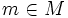$m \in M$ and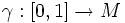$\gamma:[0,1]\to M$ be a loop at$m$ (viz$\gamma(0) = \gamma(1) = m$. The holonomy of$\gamma$ is defined as the following linear transformation at$E_p$: it sends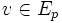$v \in E_p$ to the element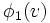$\phi_1(v)$ where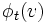$\phi_t(v)$ is the transport of$v$ along$\gamma$, with respect to the connection$\nabla$.